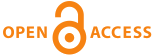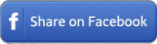Home|Journals|Articles by YearDirectory for Medical Articles in any field in Title in Summary in Keywords in Authors in journals

 Original ResearchJECAS. 2016; 3(2): 30-38

#### The Photogravitational Circular Restricted Four-body Problem with Variable Masses

Abdullah A. Ansari.

##### Abstract

In this paper, we examine the stability of the equilibrium points in the photogravitational circular restricted four-body problem by considering all the masses are varying with time, one of the three masses are taken as source of radiation pressure and all the masses are placed at the vertices of an equilateral triangle. We derive the equations of motion under the effect of solar radiation pressure by using the Meshcherskii transformations. We have plotted the equilibrium points, time series, surfaces of motion of infinitesimal body, Poincare surface of sections and Newton-Raphson basin of attraction. Here five equilibrium points are found but it is ten in the classical case. From the time series, it is observed that the orbit will not be periodic. The surfaces of motion of the infinitesimal body are studied in the space. The Poincare surface of section is in the discrete type of pattern. The convergence of the equilibrium points is studied by the Newton-Raphson basin of attraction. Finally, we have examined the stability of the equilibrium points and found that all the equilibrium points are unstable.

Key words: Radiation Pressure; variable masses; equilateral triangle; Poincare surface of sections; Newton-Raphson basin of attraction.

Full-text options

 Share this ArticleOnline Article Submission
• ejmanager.com